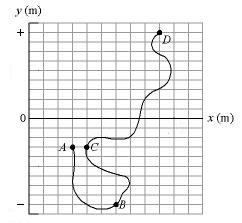# Difficult Vectors Problem

## Homework Statement

The figure below gives the path of a squirrel moving about on level ground, from point A (at time t = 0), to points B (at t = 6.00 min), C (at t = 12.0 min), and finally D (at t = 18.0 min). Both axes are marked in increments of 1.50 m (therefore the diagram is not drawn to scale). Consider the average velocities of the squirrel from point A to each of the other three points.(a) Of the three average velocities, which has the least magnitude?
What is this average velocity in magnitude-angle notation?
(b) Which has the greatest magnitude?
What is this average velocity in magnitude-angle notation?

## The Attempt at a Solution

OK. but what do you mean by magnitude-angle notation?

Not sure, thats what is asked for ....
the answer boxes look like this
[blank] m/s, [blank] ° (counterclockwise from the positive x axis)

Fredrik
Staff Emeritus
Gold Member
The first thing you need to do is post the definitions of "magnitude" and "average velocity". The second thing is to try to use those definitions to answer the questions.

Student100
Gold Member

## Homework Statement

The figure below gives the path of a squirrel moving about on level ground, from point A (at time t = 0), to points B (at t = 6.00 min), C (at t = 12.0 min), and finally D (at t = 18.0 min). Both axes are marked in increments of 1.50 m (therefore the diagram is not drawn to scale). Consider the average velocities of the squirrel from point A to each of the other three points.(a) Of the three average velocities, which has the least magnitude?
What is this average velocity in magnitude-angle notation?
(b) Which has the greatest magnitude?
What is this average velocity in magnitude-angle notation?